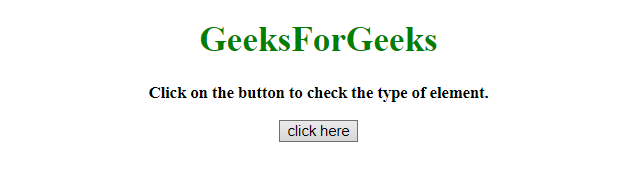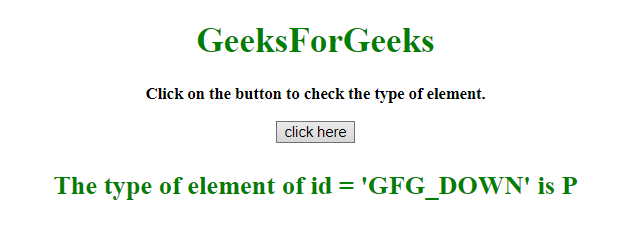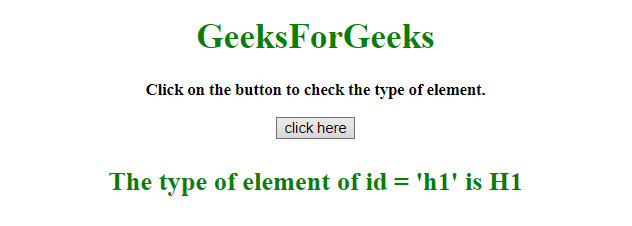# How to get the type of DOM element using JavaScript?

• Last Updated : 12 Sep, 2019

The task is to get the type of DOM element by having its object reference. Here we are going to use JavaScript to solve the problem.
Approach 1:

• First take the reference of the DOM object to a variable(Here, In this example an array is made of IDs of the element, then select random ID and select that particular element).
• Use .tagName property to get the element name.

Example 1: This example using the appraoach discussed above.

Hey geek! The constant emerging technologies in the world of web development always keeps the excitement for this subject through the roof. But before you tackle the big projects, we suggest you start by learning the basics. Kickstart your web development journey by learning JS concepts with our JavaScript Course. Now at it's lowest price ever!

 ```<``html``>`` ` `<``head``>``    ``<``title``>``        ``How to get the type of DOM ``      ``element using JavaScript?``    ``````<``center``>`` ` `    ``<``body` `style``=``"text-align:center;"``>``        ``<``h1` `id``=``"h1"` `style``=``"color:green;"``>  ``        ``GeeksForGeeks  ``    ````        ``<``p` `id``=``"GFG_UP"` `           ``style="font-size: 15px;``                  ``font-weight: bold;">``        ````        ``<``button` `id``=``"button"` `onclick``=``"GFG_Fun()"``>``            ``click here``        ````        ``<``p` `id``=``"GFG_DOWN"` `           ``style="font-size: 24px; ``                  ``font-weight: bold; ``                  ``color: green;">``        ````        ``<``script``>``            ``var up = document.getElementById('GFG_UP');``            ``var down = document.getElementById('GFG_DOWN');``            ``var arr = ["h1", "GFG_UP", "button", "GFG_DOWN"];``            ``up.innerHTML =``              ``"Click on the button to check the type of element.";`` ` `            ``function GFG_Fun() {``                ``var id = arr[Math.floor(Math.random() * arr.length)];``                ``down.innerHTML = ``                  ``"The type of element of id = '" + id + "' is " ``                ``+ document.getElementById(id).tagName;``            ``}``        ````    `````` ` ``

Output:

• Before clicking on the button:• After clicking on the button:Approach 2:

• First take the reference of the DOM object to a variable(Here, In this example an array is made of IDs of the element, then select random ID from the array and select that particular element).
• Use .nodeName property to get the element name.

Example 2: This example using the approach discussed above.

 ```<``html``>`` ` `<``head``>``    ``<``title``>``        ``How to get the type of ``      ``DOM element using JavaScript?``    ``````<``center``>`` ` `    ``<``body` `style``=``"text-align:center;"``>``        ``<``h1` `id``=``"h1"` `style``=``"color:green;"``>  ``        ``GeeksForGeeks  ``    ````        ``<``p` `id``=``"GFG_UP"` `           ``style="font-size: 15px;``                  ``font-weight: bold;">``        ````        ``<``button` `id``=``"button"` `onclick``=``"GFG_Fun()"``>``            ``click here``        ````        ``<``p` `id``=``"GFG_DOWN"``           ``style="font-size: 24px; ``                  ``font-weight: bold; ``                  ``color: green;">``        ````        ``<``script``>``            ``var up = document.getElementById('GFG_UP');``            ``var down = document.getElementById('GFG_DOWN');``            ``var arr = ["h1", "GFG_UP", "button", "GFG_DOWN"];``            ``up.innerHTML = ``              ``"Click on the button to check the type of element.";`` ` `            ``function GFG_Fun() {``                ``var id = arr[Math.floor(Math.random() * arr.length)];``                ``down.innerHTML =``                  ``"The type of element of id = '" + id + "' is " ``                ``+ document.getElementById(id).nodeName;``            ``}``        ````    `````` ` ``

Output:

• Before clicking on the button:• After clicking on the button:My Personal Notes arrow_drop_up